# Fourier Transform Notes | Study Signals and Systems - Electrical Engineering (EE)

## Electrical Engineering (EE): Fourier Transform Notes | Study Signals and Systems - Electrical Engineering (EE)

The document Fourier Transform Notes | Study Signals and Systems - Electrical Engineering (EE) is a part of the Electrical Engineering (EE) Course Signals and Systems.
All you need of Electrical Engineering (EE) at this link: Electrical Engineering (EE)

Fourier Transform

A representation for aperiodic signals

We have already seen that a broad class of functions (which satisfy the Dirichlet's conditions) can be written in the form of Fourier series .That is ,for a periodic function x(t) satisfying the Dirichlet's conditions , we may say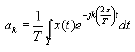where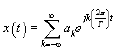Although this covers a broad class of functions, it puts a serious restriction on the function. That is, Periodicity . So the next question that naturally pops up in one's mind is , "Can we extend our idea of the Fourier series so as to include non periodic functions ?" . This precisely is our goal for this part , the basic inspiration being, an aperiodic signal may be looked at as a periodic signal with an infinite period. Note what follows is not a mathematically rigourous excercise, but will help develop an intuition for the Fourier Transform for aperiodic signals.

The Fourier Transform

Let's start with a simple example .Consider a following function, periodic with period T.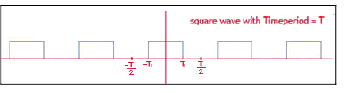Clearly this is our familiar square wave . Let's see what happens to its Frequency domain representation as we let T to approach infinity . We know that the Fourier coefficients of the above function can be written as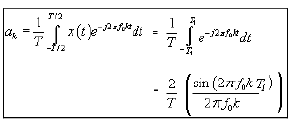where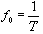= 1. For T = 4, the expression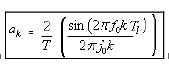reduces to :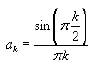Now we know that every value of k in this equation gives us the coefficient corresponding to the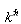multiple of the fundamental frequency of the signal. Let's plot the Frequency Domain representation of the signal, which we shall also call the spectrum of the signal.
Note the horizontal axis represents frequency, although the points marked indicate only scale.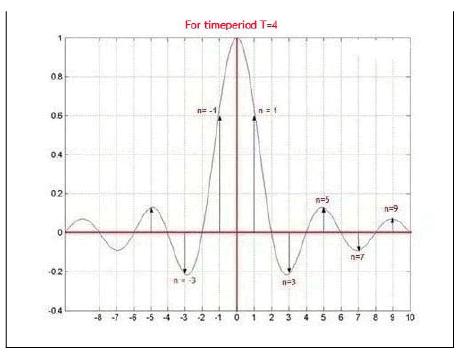Now let's double the time period .the expression for the Fourier coefficients will become :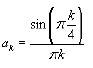Since frequency is reciprocal of time period,

as the time period T increases, the distance between the consecutive frequencies f0 in the spectrum will reduce ,and we'll get the following plot.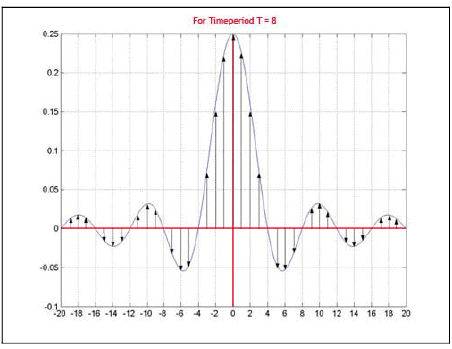If we reduce the natural frequency by another half ( that is increase the time period by a factor of two ) , we'll get the following frequency spectrum :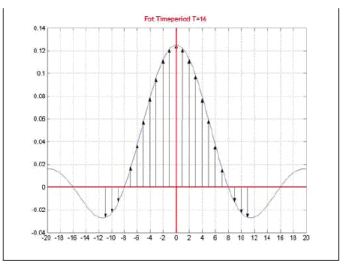Notice as the period of the periodic signal is increased, the spacing between adjacent frequency components decreases.

Finally when the period of the signal tends to infinity , i.e. the signal is aperiodic, the frequency spectrum becomes a continuous.
By looking at the plots we can infer that as we will increase the time period more and more, we'll get more and more closely placed frequencies in the spectrum, i.e.: complex exponentials with closer and closer frequencies are required to represent the signal. Hence if we let T to approach infinity we'll get frequency infinitesimally close to each other. This is same as saying that we'll get every possible frequency and hence the whole continuous frequency axis. Thus our frequency spectrum will no more be a series , but will be a continuous function. The representation will change from a summation over discrete frequencies to an integration over the entire frequency axis. The function, which (like the Fourier Series coefficients) gives what is crudely the strength of each complex exponential in the representation is formally called the Fourier Transform of the signal. The representation takes the form: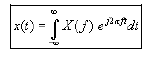where X(f) is the Fourier Transform of x(t). Note the similarity of the above equation with the Fourier Series summation in light of the preceding discussion.
This equation is called the Inverse Fourier Transform equation, x(t) being called the Inverse Fourier Transform of X(f)
Such a representation for an aperiodic signal exists, of course subject to some conditions, but we'll come to those a little later.

The Fourier Transform equation

The Fourier Transform of a function x(t) can be shown to be: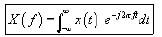This equation is called the Fourier Transform equation.
(As a convention, we generally use capital letters to denote the Fourier transform)
Obviously not in all cases are we guaranteed that the integral on right hand side will converge.
We'll next discuss the conditions for the Fourier Transform of an aperiodic signal to exist.

Recap:
Under certain conditions, an aperiodic signal x(t) has a Fourier transform X(f) and the two are related by: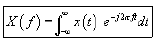(Fourier Transform equation)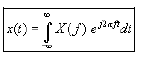( Inverse Fourier Transform equation)

Now, lets go on to the conditions for existence of the Fourier Transform. Again notice the similarity of these conditions with the Dirichlet conditions for periodic signals.

Dirichlet Conditions for convergence of Fourier Transform

Consider an aperiodic signal x(t). Its Fourier Transfrom exists (i.e the Transform integral converges) and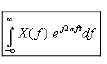converges to x(t), except at points of discontinuity provided:

1) x(t) is absolutely integrable . i.e: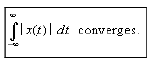2) x(t) has only a finite number of extrema in any finite interval.

For example,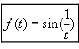does not satisfy this condition in, say (0,1).

3 ) x(t) has only a finite number of discontinuities in any finite interval. For example the following function (the so called Dirichlet's function ) will not satisfy this condition.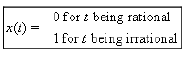These 3 conditions satisfied,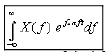will converge to x(t) at all points of continuity of x(t). At points of discontinuity of x(t), this integral converges to the average of the left hand limit and the right hand limit of x(t) at that point.

Conclusion:

In this lecture you have learnt:

• An aperiodic signal may be looked at as a periodic signal with an infinite period .
• We learnt what inverse Fourier transform is & derived its equation.
• We saw Dirichlet Conditions for convergence of Fourier Transform.
The document Fourier Transform Notes | Study Signals and Systems - Electrical Engineering (EE) is a part of the Electrical Engineering (EE) Course Signals and Systems.
All you need of Electrical Engineering (EE) at this link: Electrical Engineering (EE)Use Code STAYHOME200 and get INR 200 additional OFF

## Signals and Systems

40 videos|41 docs|25 tests

Track your progress, build streaks, highlight & save important lessons and more!

,

,

,

,

,

,

,

,

,

,

,

,

,

,

,

,

,

,

,

,

,

;